# A Simple Method to Assess AFE Performance in your Communication SoC

By Manuel Mota, Product Marketing Manager, Synopsys

System designers need Analog Front-End (AFE) IP that can easily integrate into their SoC with less risk. Performance requirements have become even more challenging with the ever evolving technologies in smarter systems. Selecting an AFE IP and its components [Analog-to-Digital Converters (ADCs), Digital-to-Analog Converters (DACs) and Phase-Locked Loops (PLLs)] to meet SoC requirements for processing broadband signals is complex and can lead to over-specification and inefficient power consumption.

A simplified method is needed to determine if the electrical characteristics of any given AFE are adequate for the targeted application, such as broadband communications, in the context of wireless or wireline connectivity, cellular communications, and digital TV reception. Additionally, system designers need a tool to explore tradeoffs between relative performance and operating modes of different components to find the optimal performance, power, area and cost for their SoCs.

## The Analog Front-End

In a broadband signal transceiver digital SoC, the AFE translates analog signals into digital signals for further digital processing and vice versa for transmission purposes. The AFE also doubles as the analog interface for communication between the digital SoC and the analog RF transceiver chip. Figure 1 illustrates an AFE example in a digital baseband chip.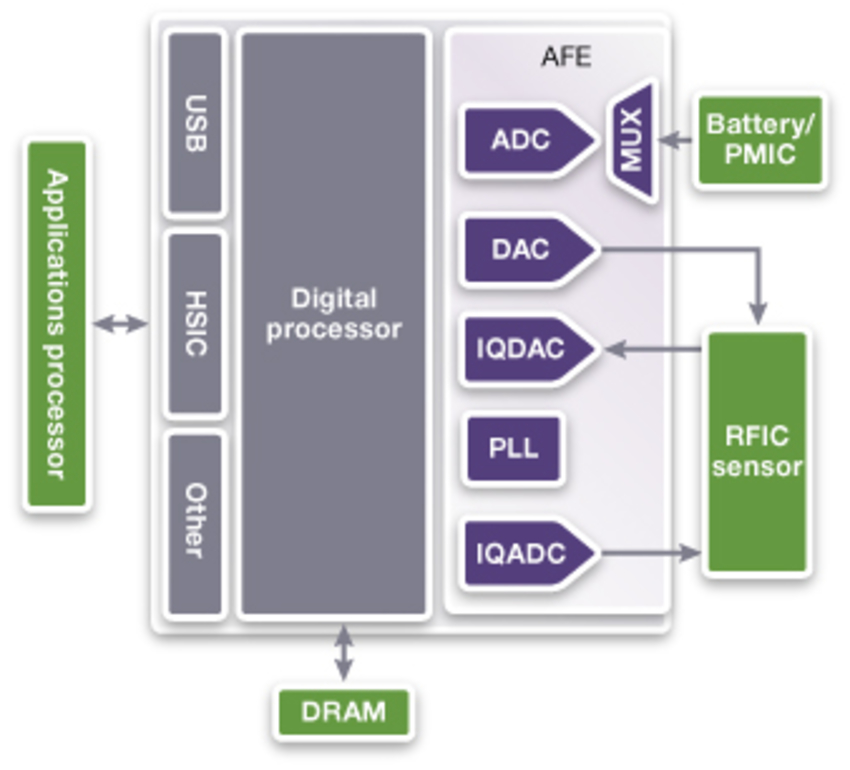Figure 1: AFE in a digital baseband chip

## Defining AFE Performance Contributions

Determining the AFE performance contribution to the total transceiver signal-to-noise ratio (SNR) performance, takes into account key characteristics of the signal being processed (input signal bandwidth and amplitude, modulation scheme) and the quality of the AFE components' performance (ADC SNR, PLL clock jitter, etc.).

Although the method outlined here focuses on the ADC (reception) impact, the same applies to DAC (transmission). To determine the data converter's intrinsic SNR contribution to the system Error Vector Magnitude (EVM), start with the ADC SNR specification (SNRnyq). The SNRnyq specification (the intrinsic SNR) is a measure of the ratio between the signal power (signal is assumed to be a full scale pure sine wave) and the power of all noise contributions intrinsic to the ADC. This includes thermal noise and quantization noise, integrated across the complete ADC Nyquist band.

However, the effective converter SNR can be improved by tuning signal characteristics such as the signal bandwidth and the input signal amplitude, as well as the contribution of PLL clock jitter.

## Accounting for Input Signal Bandwidth

Modern frequency domain digital signal processing techniques are applied to demodulate the signal at the ADC output. However, only the signal contents within the bandwidth (BW) of interest are considered such that all out-of-band noise components are effectively filtered out.

Designers can improve data converter SNR performance by spreading the total noise power it generates through a larger frequency spectrum, thereby increasing the converter sampling rate (Fs) beyond the minimum Nyquist limit. This leads to a lower noise power density at any given band, resulting in an improved SNR when only the signal band of interest is considered - a technique called oversampling.

Oversampling brings additional benefits of simplifying the analog, anti-aliasing, filtering at the input of an ADC or the reconstruction filter at the output of a DAC. This is due to the signal images, centered in multiples of the sampling frequency, having larger frequency separation.

#### Accounting for Input Signal Amplitude

The main contributors to data converters' intrinsic noise are quantization noise and thermal noise, typically assumed to be white noise with a uniform power distribution. The power of these noise components is mainly independent of the signal amplitude. Therefore any reduction (back-off) of full-scale signal amplitude leads to a reduction of the effective SNR.

In communication systems, the signal often uses complex modulation schemes with a large Peak-to-Average Ratio (PAR). To saturate the ADC, which may result in signal clipping, higher distortion and out-of-band power, the signal has to be backed-off (attenuated) such that the peak of the signal falls within the ADC full scale range.

Additional back-off may be required to prevent saturation due to normal signal strength variations, transmitted over a non-ideal channel, or to take into account the strength of adjacent channels that may not have been completely filtered prior to the ADC input.

The signal back-off (IBO) takes into account several impairments:

• Presence of strong out-of-band signals that were not adequately filtered
• Variations of the radio signal strength that are not compensated by the transceiver automatic gain control
• Gain inaccuracy in the analog signal chain due to Process-Voltage-Temperature (PVT) variations

## Determining PLL Clock Jitter Contribution

The uncertainty on the sampling instant due to PLL clock jitter (σLTJ) can also impact the data converter SNR performance.

For complex modulated signals such as OFDM (Orthogonal Frequency-Division Multiplexing), the sampling clock inter-modulates with each sub-carrier, resulting in the convolution of the clock phase noise with each sub-carrier scaled by its respective frequency and amplitude. Figure 2 demonstrates the result of this convolution in the frequency domain. By comparison, quantization and thermal noise add a uniform power noise density across the spectrum (blue area in Figure 2).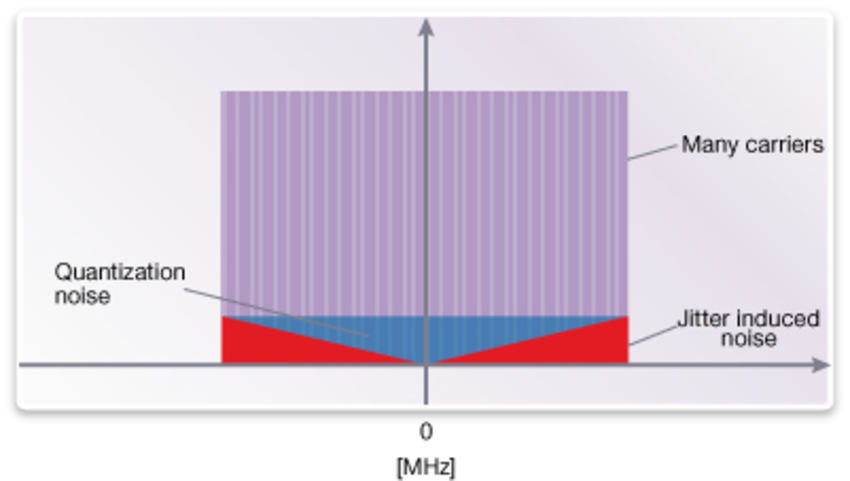Figure 2: Effect of clock jitter in a OFDM signal

## Calculating AFE Performance Contributions

The following equations summarize the procedure to determine the total contribution of the AFE to the system level SNR.

Step 1: Calculate the contribution of the data converter to the system SNR.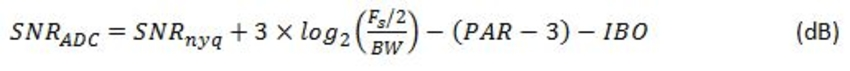SNRnyq,PAR and IBO (in units of dB and Fs); signal bandwidth (BW) (in units of Hz)

Step 2: Calculate the contribution of the PLL clock jitter to the system SNR.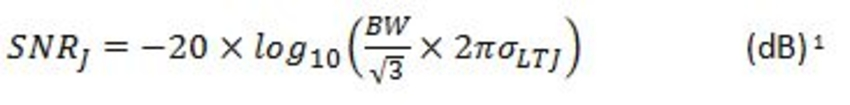1 This equation assumes the signal is a baseband signal, spanning from 0 Hz to BW.

BW (units of Hz); σLTJ (in units of seconds)

Step 3: Assume a few dBs as the typical contribution to system SNR due to un-compensated I and Q-imbalances.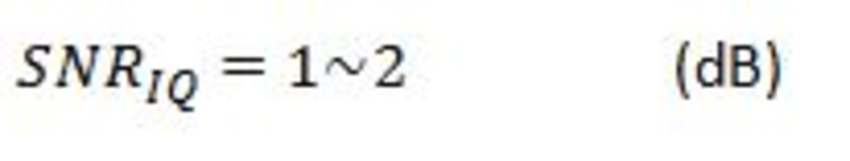Step 4: The total contribution of the AFE to the overall system SNR is the sum of these three contributions.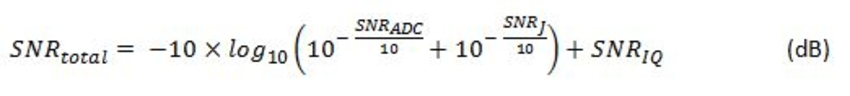Step 5: Determine the AFE contribution to system level EVM. EVM is the parameter typically used to measure the quality of the digital transceiver.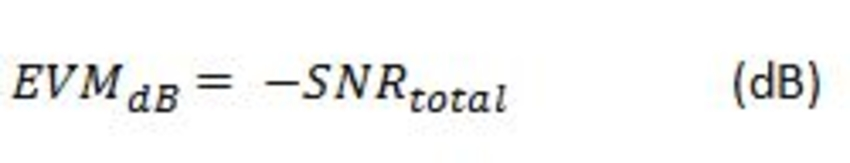A high-performing AFE is one where its EVM contribution only marginally impacts the total transceiver performance. An impact of 0.5 to 0.7 dB is often acceptable.

## Applying the Method to the AFE on a WiFi 802.11ac Transceiver

To apply these calculations, consider a transmission system where the signal is OFDM modulated with a BW of 160MHz and each sub-carrier modulated with a QAM256 modulation scheme (this is similar to a WiFi 802.11ac transceiver). In addition, consider the implementation of a Zero-IF demodulation scheme.

In this case, the resulting baseband quadrature demodulated signal at the input of I and Q ADC has, respectively, a channel bandwidth of 80MHz.

Further assuming the following characteristics of the AFE:

• ADC SNR = 62 dB (SNRnyq)
• ADC sampling rate = 160 MSPS (Fs)
• Clock long term jitter = 8 ps-rms (σLTJ)
• OFDM signal peak to average ratio = 12 dB (PAR)
• Signal back-off = 10 dB (IBO)
• ADC signal BW = 80 MHz (BW)

Then, the overall SNR of the AFE is:

• SNRJ = 52.7 dB
• SNRtotal = 42.6 dB

In this example, the EVM requirements to demodulate a QAM256 signal are in the order of -33.8dB, (required SNR is 33.8dB). There is a margin of ~8.8dB to the required SNR, resulting in an acceptable degradation of only 0.6 dB of the total system performance.

Similar SNRtotal can be achieved with an AFE with the following characteristics:

• ADC SNR = 66 dB (SNRnyq)
• Clock long term jitter = 20 ps-rms (σLTJ)

It is therefore possible to trade-off clock jitter with ADC performance to achieve the same goal.

## Conclusion

With the method outlined here, system designers can quickly determine if the electrical characteristics of any given AFE are adequate for their targeted application, such as broadband communications in the context of wireless or wireline connectivity, cellular communications and digital TV reception. Using this method, system designers can quickly estimate the impact of the AFE performance in the system and understand if it matches their SoC requirements, thus avoiding over-specification and inefficient power consumption. In addition, designers can quickly assess different alternatives and configuration tradeoffs to find the optimal performance, power, area and costs for their SoCs.